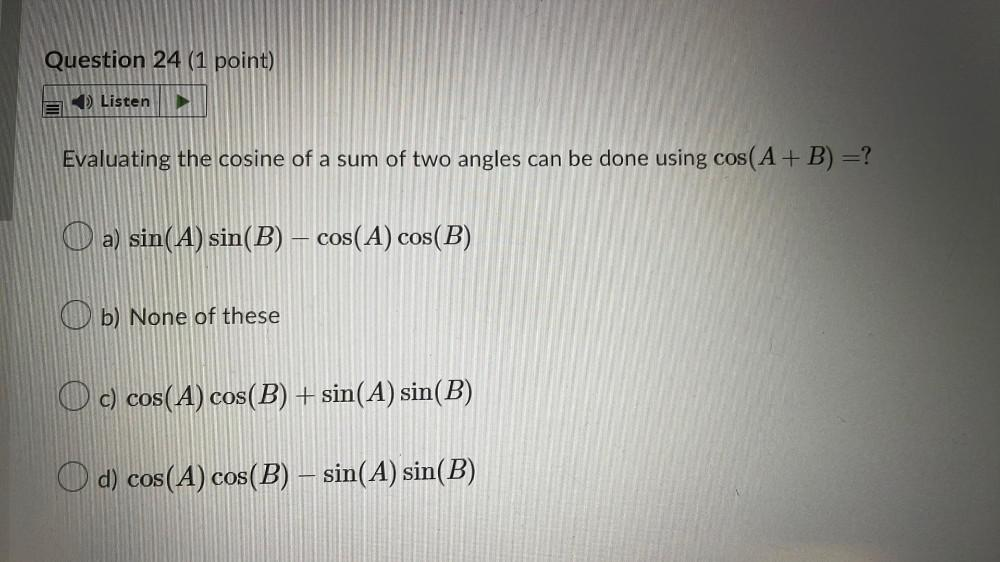Question:

# Evaluating the cosine of a sum of two angles can be done

Last updated: 7/31/2022Evaluating the cosine of a sum of two angles can be done using cos(A + B) = ? a) sin(A) sin(B) – cos(A) cos(B) b) None of these c) cos(A) cos(B) + sin(A) sin(B) d) cos(A) cos(B) – sin(A) sin(B)